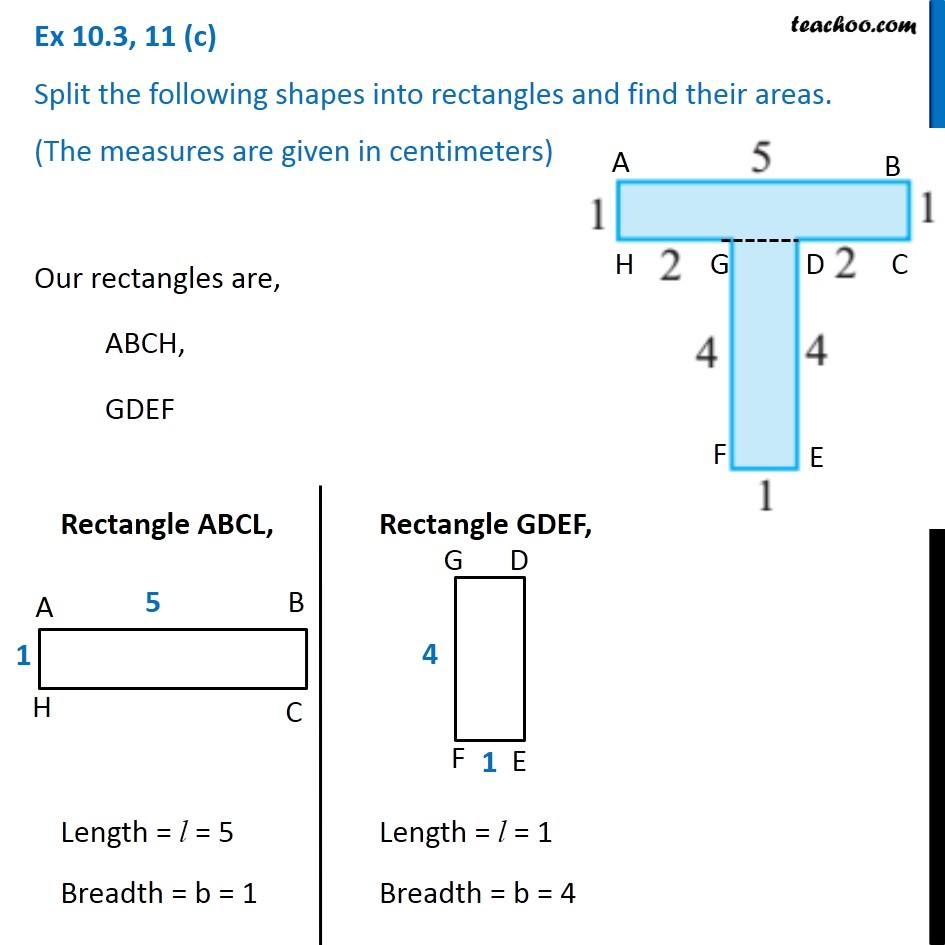Ex 10.3

Chapter 10 Class 6 Mensuration
Serial order wiseLearn in your speed, with individual attention - Teachoo Maths 1-on-1 Class

### Transcript

Ex 10.3, 11 (c) Split the following shapes into rectangles and find their areas. (The measures are given in centimeters) Our rectangles are, ABCH, GDEF Rectangle ABCL, Length = l = 5 Breadth = b = 1 Rectangle GDEF, Length = l = 1 Breadth = b = 4 Area = l × b = 5 × 1 = 5 〖𝑐𝑚〗^2 Area = l × b = 1 × 4 = 5 〖𝑐𝑚〗^2 Area of given shape = 5 + 4 = 9 cm2